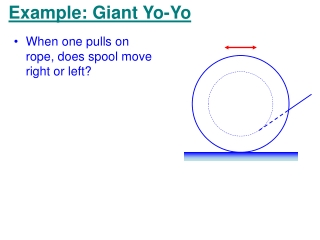DownloadDownload PresentationExample: Giant Yo-Yo

Example: Giant Yo-Yo

Télécharger la présentationExample: Giant Yo-Yo

- - - - - - - - - - - - - - - - - - - - - - - - - - - E N D - - - - - - - - - - - - - - - - - - - - - - - - - - -
Presentation Transcript

1. Example: Giant Yo-Yo • When one pulls on rope, does spool move right or left?

2. Example: Giant Yo-Yo • When one pulls on rope, does spool move right or left? • Depends on torque about pivot point (contact with floor)

3. Example: Giant Yo-Yo • When one pulls on rope, does spool move right or left? • Depends on torque about pivot point (contact with floor) • If rope projects to a point behind pivot, rolls right

4. Example: Giant Yo-Yo • When one pulls on rope, does spool move right or left? • Depends on torque about pivot point (contact with floor) • If rope projects to a point behind pivot, rolls right • If rope projects in front, rolls left

5. Torque as a Vector • Torque is a vector • Magnitude • What is direction? • Define torque vector with cross product of position and force vectors

6. The Vector Cross Product • If C = A x B, then • C = AB sinq • Direction of C given by Right Hand Rule • Properties

7. The Vector Cross Product • Unit Vectors and the algebraic form of cross product

8. Angular Momentum • Consider the torque on a particle • Call L = r x p the angular momentum (of the particle about the origin)

9. Example: Airplane Overhead • What is the angular momentum of a plane flying overhead? • It’s not zero! • Even if plane is flying level • Note that L has a constant magnitude with a direction into the page v q h r

10. Angular Momentum • Now consider a system of particles • Like force and momentum, the net torque gives the rate of change of angular momentum

11. Spinning Rigid Objects • What’s the angular momentum of a spinning, rigid object? • Always measure from (and perpendicular to) axis of rotation (which is along z axis)

12. Spinning Rigid Objects • What happens when we apply a torque to a spinning object?

13. Translational-Angular Analogs

14. Conservation of Angular Momentum • If there are no external torques, angular momentum cannot change! • Like linear momentum, angular momentum is conserved • If moment-of-inertia changes, so does angular velocity!

15. Example: Person on Stool d • If a person is spinning on a stool holding weights, they spin faster if they pull them in

16. Example: Person on Stool d • L is conserved, is kinetic energy?

17. Example: Person on Stool d • Where did energy come from? • Work required to pull in weights!

18. Example: P with Wheel on Stool • What happens when the wheel in the picture is turned over? • Since L is conserved, but wheel’s L changes sign, the person on the stool must start spinning to make up the difference! • But which way?

19. Gyroscopes and Tops • Torque is a vector! • Examples (pulleys, etc) have had t and L parallel • What if they’re not parallel? • What ifrandLare parallel? Then we have a gyroscope or a top.

20. Gyroscopes and Tops • Find t and L for top • Now, what is dL? • So the L vector moves perpendicular to itself. • It doesn’t change magnitude, so it must sweep out a circle • Call this precession

21. Gyroscopes and Tops • How fast is the precession? f

22. Gyroscopes and Tops • Consider spinning gyroscope held horizontally • L is horizontal • When end is dropped will begin to precess, giving L a vertical component • Where does this component come from? There’s no verticalt! • In fact end will bob up and down so that there is never any vertical L

23. Animation2 Animation2 Animation1

24. As an example of the usefulness of gyroscopes, suppose you are in a spacecraft in deep space and you need to alter your trajectory. You need to turn the spacecraft around in order to fire the engines in the correct direction. But how do you turn a spacecraft around in empty space?

25. Rotation about Arbitrary Axis  • Consider rotating stick with masses • To keep it spinning about this axis, a torque must be applied • Thus L not constant, but spins around • What about L=Ia? • In fact I is a tensor (like a vector, but with two spatial indices). L t